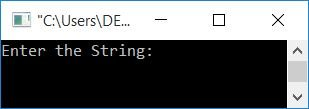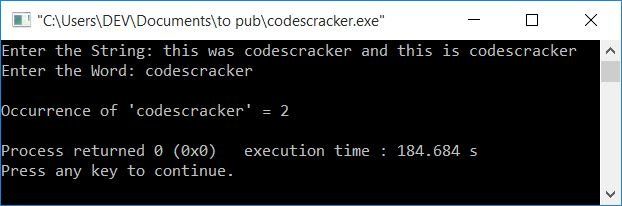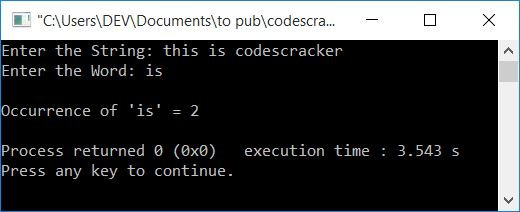# C++ program to count the occurrences of a word in a string

In this article, you will learn and get code to count occurrences of a word in a string in C++. Here is the list of programs available:

## Determine the Frequency of a Word in a String

This program counts the occurrence of a word in a given string. But this program is best suited to find the frequency of a sub-string (without space) in a string (entered by the user). I'll tell you about the reason later on.

The question is: write a program in C++ that finds the frequency of a word (a sub-string) in a string. Here is its answer:

```#include<iostream>
#include<stdio.h>
using namespace std;
int main()
{
char str, word;
int i=0, j, temp, countW=0, chk;
cout<<"Enter the String: ";
gets(str);
cout<<"Enter the Word: ";
gets(word);
while(str[i]!='\0')
{
temp = i;
j=0;
while(word[j]!='\0')
{
if(str[i]==word[j])
i++;
j++;
}
chk = i-temp;
if(chk==j)
countW++;
i = temp;
i++;
}
cout<<"\nOccurrence of '"<<word<<"' = "<<countW;
cout<<endl;
return 0;
}```

This program was built and runs under the Code::Blocks IDE. Here is its sample run:Now supply any string, say this was codescracker and this is codescracker, and then a word, say codescracker to find the frequency or occurrence of the word codescracker in the string. Here is the final snapshot of the sample run:The dry run of the above program with user inputs of "this was codescracker and this is codescracker" as a string and "codescracker" as a word goes like this:

• i = 0, countW = 0 are the initial values.
• When the user enters the string, then it gets stored in str in a way that:
• str=t
• str=h
• str=i
• and so on up until
• str=r
• Similarly, the entered word, say codescracker gets stored in the word in this way:
• word=c
• word=o
• word=d
• and so on up until
• word=r
• Because the maximum length of a string and a word, str[] and word[], are 200 and 20, respectively. However, the entered string and word are only 46 and 12, respectively. So a null terminated character (\0) automatically gets assigned after the last character's index in both strings and words.
• Now that the while loop's condition, str[i]!='\0' or str!='\0' or t!='\0', evaluates to true, program flow enters the loop.
• And either i or 0 is set to temp. So temp=0 and j=0
• The condition, word[j]!='\0' or word!='\0' or c!='\0' evaluates to be true, therefore program flow goes inside the loop.
• And the condition of if, that is str[i]==word[j] or str==word or t==c evaluates to be false, therefore program flow does not go inside its body (if's body). So it just increments the value of j and goes back and evaluates the inner while loop's condition again.
• The process of evaluating this while loop continues until its condition evaluated to be false.
• When its condition evaluates to false, the value of i will be 0 and j = 12 after exiting this loop for the time being.
• Now, i-temp, 0-0, or 0 is set to chk.
• And the condition chk==j or 0==12 evaluates to be false; therefore, program flow does not go inside its (if's) body.
• Now the value of temp (0) gets initialized to i. So i=0
• And finally, the value of i gets incremented. So i=1
• Program flow goes back and evaluates the condition of the while loop again.
• This process continues until the condition of the outer while loop evaluates to be false.
• On continuing the evaluation, here are the values we'll get after each evaluation:
• temp=0 (before inner while), j=0 (before inner while), i=0 (after inner while), j=12 (after inner while), countW=0, i=1 (final)
• temp=1 (before inner while), j=0 (before inner while), i=1 (after inner while), j=12 (after inner while), countW=0, i=2 (final)
• Similarly temp=2, j=0, i=2, j=12, countW=0, i=3
• temp=3, j=0, i=4, j=12, countW=0, i=4
• temp=4, j=0, i=4, j=12, countW=0, i=5
• temp=5, j=0, i=5, j=12, countW=0, i=6
• temp=6, j=0, i=7, j=12, countW=0, i=7
• temp=7, j=0, i=8, j=12, countW=0, i=8
• temp=8, j=0, i=8, j=12, countW=0, i=9
• temp=9, j=0, i=21, j=12, countW=1, i=10
• temp=10, j=0, i=21, j=12, countW=1, i=11
• and so on upto
• temp=45, j=0, i=46, j=12, countW=2, i=46
• Now print the value of countW as output; that will be the result.

### Modified Version of the Previous Program

If your only goal is to count the occurrences of a word (without regard for the entire word) in a given string, the previous program is correct; otherwise, if you want to find the frequency of a single word in a string, the above program has a limitation, which is listed below.

Important: The above program has the limitation that it increments the value of countW if the entered word appears in any other word in the string. For example, if the string is "this is codescracker" and the entered word is "is," Therefore, this also gets counted.

Here is the snapshot of the sample run (of the previous program) that clarifies what I mean to say:So to improve the previous program, here is the modified version of that program:

```#include<iostream>
#include<stdio.h>
#include<string.h>
using namespace std;
int main()
{
char str, word;
int i, j, ls, lw, k, countWord=0, chk, doIncrement;
cout<<"Enter the String: ";
gets(str);
cout<<"Enter the Word: ";
gets(word);
ls = strlen(str);
lw = strlen(word);
for(i=0; i<ls; i++)
{
k = i;
doIncrement = 0;
for(j=0; j<lw; j++)
{
if(str[i]==word[j])
{
if(k>0 && (k+lw)<ls)
{
if(str[k-1]== ' ' && str[k+lw]==' ')
doIncrement=1;
}
else if(k==0 && (k+lw)<ls)
{
if(str[k+lw]==' ')
doIncrement=1;
}
else if(k>0 && (k+lw)==ls)
{
if(str[k-1]== ' ')
doIncrement=1;
}
if(doIncrement==1)
i++;
else
break;
}
}
chk = i-k;
if(chk==lw)
countWord++;
i = k;
}
cout<<"\nOccurrence of '"<<word<<"' = "<<countWord;
cout<<endl;
return 0;
}```

Here is its sample run:C++ Quiz

« Previous Program Next Program »